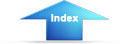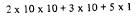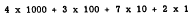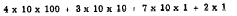Custom SearchIntroduction to Mathematics and AlgebraDEFINITIONS AND SYMBOLSCHAPTER 1

NUMBER SYSTEMS AND SETS

Mathematics is a basic tool. Some use of mathematics is found in every rating in the Navy, from the simple arithmetic of counting for inventory purposes to the complicated equations encountered in computer and engineering work. Storekeepers need mathematical computation in their bookkeeping. Damage Controlmen need mathematics to compute stress, centers of gravity, and maximum permissible roll.  Electronics principles are frequently stated by means of mathematical formulas. Navigation and engineering also use mathematics to a great extent. As maritime warfare becomes more and more complex, mathematics achieves ever increasing importance as an essential tool. From the point of view of the individual there are many incentives for learning the subject.  Mathematics better equips him to do his present job. It will help him in attaining promotions and the corresponding pay increases. Statistically it has been found that one of the best indicators of a mans potential success as a naval officer is his understanding of mathematics. This training course begins with the basic facts of arithmetic and continues through some of the early stages of algebra. An attempt is made throughout to give an understanding of why the rules of mathematics are true. This is done because it is felt that rules are easier to learn and remember if the ideas that led to their development are understood.

Many of us have areas in Our mathematics background that are hazy, barely understood, or troublesome. Thus, while it may at first seem beneath your dignity to read chapters on fundamental arithmetic, these basic concepts may be just the spots where your difficulties lie. These chapters attempt to treat the subject on an adult level that will be interesting and informative.

COUNTING

Counting is such a basic and natural process that we rarely stop to think about it. The process is based on the idea of ONE-TO-ONE CORRESPONDENCE, which is easily demonstrated by using the fingers. When children count on their fingers, they are placing each finger in one-to-one correspondence with one of the objects being counted. Having outgrown finger counting, we use numerals.

NUMERALS

Numerals are number symbols. One of the simplest numeral systems is the Roman numeral system, in which tally marks are used to represent the objects being counted. Roman numerals appear to be a refinement of the tally method still in use today. By this method, one makes short vertical marks until a total of four is reached; when the fifth tally is counted, a diagonal mark is drawn through the first four marks. Grouping by fives in this way is reminiscent of the Roman numeral system, in which the multiples of five are represented by special symbols.

A number may have many names." For example, the number 6 may be indicated by any of the following symbols: 9 - 3, 12/2, 5 + 1, or 2 x 3. The important thing to remember is that a number is an idea; various symbols used to indicate a number are merely different ways of expressing the same idea.

POSITIVE WHOLE NUMBERS

The numbers which are used for counting in our number system are sometimes called natural numbers. They are the positive whole numbers, or to use the more precise mathematical term, positive INTEGERS. The Arabic numerals from 0 through 9 are called digits, and an integer may have any number of digits. For example, 5, 22, and 7,049 are all integers. The number of digits in an integer indicates its rank; that is, whether it fs "in the hundreds," "in the thousands," etc. The idea of ranking numbers in terms of tens, hundreds, thousands, etc., is based on the PLACE VALUE concept.

PLACE -VALUE

Although a system such as the Roman numeral system is adequate for recording the results of counting, it is too cumbersome for purposes of calculation. Before arithmetic could develop as we know it today, the following two important concepts were needed as additions to the counting process:

1. The idea of 0 as a number.

2. Positional notation (place value).

Positional notation is a form of coding in which the value of each digit of a number depends upon its position in relation to the other digits of the number. The convention used in our number system is that each digit has a higher place value than those digits to the right of it.

The place value which corresponds to a given position in a number is determined by the BASE of the number system. The base which is most commonly used is ten, and the system with ten as a base is called the decimal system (decem is the Latin word for ten). Any number is assumed to be a base-ten number, unless some other base is indicated. One exception to this rule occurs when the subject of an entire discussion is some base other than ten. For example, in the discussion of binary (base two) numbers later in this chapter, all numbers are assumed to be binary numbers unless some other base is indicated.

DECIMAL SYSTEM

In the decimal system, each digit position in a number has ten times the value of the position adjacent to it on the right. For example, in the number 11, the 1 on the left is said to be in the "tens place, " and its value is 10 times as great as that of the 1 on the right. The 1 on the right is said to be in the "units place," with the understanding that the term "unit" in our system refers to the numeral 1. Thus the number 11 is actually a coded symbol which means "one ten plus one unit." Since ten plus one is eleven, the symbol 11 represents the number eleven. Figure l-l shows the names of several digit positions in the decimal system. If we apply this nomenclature to the digits of the integer 235, then this number symbol means "two hundreds plus three tens plus five units." This number may be expressed in mathematical symbols as follows:Notice that this bears out our earlier statement: each digit position has 10 times the value of the position adjacent to it on the right.Figure 1-l.-Names of digit positions.

The integer 4,372 is a number symbol whose meaning is "four thousands plus three hundreds plus seven tens plus two units." Expressed in mathematical symbols, this number is as follows:This presentation may be broken down further, in order to show that each digit position as IO times the place value of the position on its right, as follows:The comma which appears in a number symbol such as 4,372 is used for "pointing off" the digits into groups of three beginning at the right-hand side. The first group of three digits on the right is the units group; the second group is the thousands group; the third group is the millions group; etc. Some of these groups are shown in table l-l.

Table 1-l.-Place values and grouping.By reference to table l-l, we can verify that 5,432,786 is read as follows: five million, four hundred thirty-two thousand, seven hundred eighty-six. Notice that the word "and" is not, necessary when reading numbers of this hind.

Practice problems:

1. Write the number symbol for seven thousand two hundred eighty-one.
2. Write the meaning, in words, of the symbol
23,469.
3. If a number is in the millions, it must have
at least how many digits?
4. If a number has 10 digits, to what number
group (thousands, millions, etc.) does it belong?

1. 7,281
2. Twenty-three thousand, four hundred sixty-nine.
3. 7
4. Billions

BINARY SYSTEM

The binary number system is constructed in the same manner as the decimal system. However, since the base in this system is two, only two digit symbols are needed for writing numbers. These two digits are 1 and 0. In order to understand why only two digit symbols are needed in the binary system, we may make some observations about the decimal system and then generalize from these.

One of the most striking observations about number systems which utilize the concept of place value is that there is no single-digit symbol for the base. For example, in the decimal system the symbol for ten, the base is 10. This symbol is compounded from two digit symbols, and its meaning may be interpreted as "one base plus no units." Notice the implication of this where other bases are concerned: Every system uses the same symbol for the base, namely 10. Furthermore, the symbol 10 is not called "ten" except in the decimal system. Suppose that a number system were constructed with five as a base. Then the only digit symbols needed would be 0, 1, 2, 3, and 4. No single-digit symbol for five is needed, since the symbol 10 in a base-five system with place value means "one five plus no units." In general, in a number system using base N, the largest number for which a single-digit symbol is needed is N minus 1. Therefore, when the base is two the only digit symbols needed are 1 and O.

symbol by relating it to the decimal system. Figure l-2 shows that the place value of each digit position in the binary system is two times the place value of the position adjacent to it on the right. Compare this with figure l- 1, in which the base is ten rather than two.Figure 1-2.-Digit positions in the binary system.

Placing the digits of the number 101 in their respective blocks on figure l-2, we find that 101 means "one four plus no twos plus one unit." Thus 101 is the binary equivalent of decimal 5. If we wish to convert a decimal number, such as 7, to its binary equivalent, we must break it into parts which are multiples of 2. Since 7 is equal to 4 plus 2 plus 1, we say that it "contains" one 4, one 2, and one unit. Therefore the binary symbol for decimal 7 is 111. The most common use of the binary number system is in electronic digital computers. All data fed to a typical electronic digital computer is converted to binary form and the computer performs its calculations using binary arithmetic rather than decimal arithmetic. One of the reasons for this is the fact that electrical and electronic equipment utilizes many switching circuits in which there are only two operating conditions. Either the circuit is "on" or it is "Off )" and a two-digit number system is ideally suited for symbolizing such a situation. Details concerning binary arithmetic are beyond the scope of this volume, but are available in Mathematics, Volume 3, NavPers 10073, and Basic Electronics, NavPers 10087-A.

Practice problems:

1. Write the decimal equivalents of the binary numbers 1101, 1010, 1001, and 1111.
2. Write the binary equivalents of the decimal
numbers 12, 7, 14, and 3.

1. 13, 10, 9, and 15
2. 1100, 111, 1110, and 11

student to investigate more than one text and more than one way of approaching each new topic. At the time of printing of this course, much emphasis is being placed on so-called modern math in the public schools. Consequently, the trainee who uses this course is likely to find considerable material, in his parallel reading, which uses the ideas and terminology of the "new" math.

In the following paragraphs, a very brief introduction to some of the set theory of modern math is presented. Although the remainder of this course is not based on set theory, this brief introduction should help in making the transition from traditional methods to newer, experimental methods.Integrated Publishing, Inc. - A (SDVOSB) Service Disabled Veteran Owned Small Business